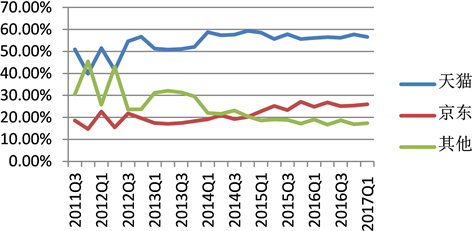﻿ 基于Lotka-Volterra模型的企业竞争战略分析

# 基于Lotka-Volterra模型的企业竞争战略分析 Competitive Strategy Analysis Based on Lotka-Volterra Model

Abstract: In this paper, we mainly use the Lotka-Volterra model in biology to learn from the interaction between population and population, and analyze the competition in the market. We think of each business as a population and construct a two-dimensional ordinary differential Lotka-Volterra model. Based on investigating the stability of the Lotka-Volterra model at the equilibrium point and analyzing the situation of enterprise competition at the equilibrium point in the market, the results provide a reference for competitive enterprises to choose competitive strategy.

1. 引言

2. 企业种群的概念

3. 模型建立

(一) 两类常微Lotka-Volterra竞争模型建立

$\left\{\begin{array}{l}\frac{\text{d}X}{\text{d}t}=\left(1-\frac{X}{{k}_{1}}-{\alpha }_{1}\cdot \frac{Y}{{k}_{2}}\right)\cdot {r}_{1}\cdot X\\ \frac{\text{d}Y}{\text{d}t}=\left(1-\frac{Y}{{k}_{2}}-{\alpha }_{2}\cdot \frac{X}{{k}_{1}}\right)\cdot {r}_{2}\cdot Y\end{array}$ (1.1)

$\left\{\begin{array}{l}\frac{\text{d}X}{\text{d}t}={r}_{1}\cdot X-{a}_{11}\cdot X\cdot X-{a}_{12}\cdot Y\cdot X\\ \frac{\text{d}Y}{\text{d}t}={r}_{2}\cdot Y-{a}_{21}\cdot X\cdot Y-{a}_{22}\cdot Y\cdot Y\end{array}$ (1.2)Table 1. The population interaction between the two mobile phone products

4. 模型结论

$\left({X}_{1},{Y}_{1}\right)=\left(0,0\right)$ , $\left({X}_{2},{Y}_{2}\right)=\left({k}_{1},0\right)$ , $\left({X}_{3},{Y}_{3}\right)=\left(0,{k}_{2}\right)$ , $\left({X}_{4},{Y}_{4}\right)=\left(a\cdot {k}_{1},b\cdot {k}_{2}\right)$

${a}_{12}>0$ 时，即企业种群Y对企业种群X的市场份额增长是抑制作用时，当 ${\alpha }_{1}>\frac{{k}_{1}}{{k}_{2}}$ 时，最终企业种群X灭绝。

${a}_{12}<0$ 时，即企业种群Y对企业种群X的市场份额增长是促进作用时，企业种群X灭绝的情况不会发生，当 ${\alpha }_{2}>\frac{{k}_{2}}{{k}_{1}}$ 时，最终企业种群Y会灭绝。

${a}_{12}>0$${a}_{21}>0$ 时， $\left({X}_{2},{Y}_{2}\right)$$\left({X}_{3},{Y}_{3}\right)$$\left({X}_{4},{Y}_{4}\right)$ 点均有可能成为稳定点，即企业种群X、Y经过市场竞争后可稳定于“企业种群Y灭绝”、“企业种群X灭绝”、“共存于当下的市场环境中”。

${a}_{12}<0$${a}_{21}<0$ 时， $\left({X}_{4},{Y}_{4}\right)$ 点有可能成为稳定点，即企业种群X、Y为互利共生关系，此时两类企业种群都不会灭绝，而是稳定于“共存于当下的市场环境中”的状态。

${a}_{12}<0$${a}_{21}>0$ 时， $\left({X}_{2},{Y}_{2}\right)$$\left({X}_{4},{Y}_{4}\right)$ 点均有可能成为稳定点，即此时企业种群X处于捕食者地位，最终可以稳定于“企业种群Y灭绝”或者“共存于当下的市场环境中”。

${a}_{12}>0$${a}_{21}<0$ 时， $\left({X}_{3},{Y}_{3}\right)$$\left({X}_{4},{Y}_{4}\right)$ 点均有可能成为稳定点，即此时企业种群Y处于捕食者地位，最终可以稳定于“企业种群X灭绝”或者“共存于当下的市场环境中”。

5. 案例分析

(一) 研究对象选取

(二) 实证分析

$\left\{\begin{array}{l}\frac{\text{d}X}{\text{d}t}={r}_{1}\cdot X-{a}_{11}\cdot X\cdot X-{a}_{12}\cdot Y\cdot X\\ \frac{\text{d}Y}{\text{d}t}={r}_{2}\cdot X-{a}_{21}\cdot X\cdot Y-{a}_{22}\cdot Y\cdot Y\end{array}$ (4.1)Table 2. Market share of Chinese B2C enterprises from 2011 to 2017Figure 1. Market share of Chinese B2C enterprises from 2011 to 2017

$\left\{\begin{array}{l}X\left(t+1\right)=X\left(t\right)\cdot \mathrm{exp}\left({r}_{1}-{\alpha }_{1}\cdot X\left(t\right)-{\beta }_{1}\cdot Y\left(t\right)\right)\\ Y\left(t+1\right)=Y\left(t\right)\cdot \mathrm{exp}\left({r}_{2}-{\alpha }_{2}\cdot Y\left(t\right)-{\beta }_{2}\cdot X\left(t\right)\right)\end{array}$ (4.2)Table 3. Eviews parameter estimation stability test of Tmall’s relative proportion (X)Table 4. Eviews parameter estimation stability test of Jingdong’s relative proportion (Y)

$\left\{\begin{array}{l}X\left(t+1\right)=X\left(t\right)\cdot \mathrm{exp}\left(0.629309-1.146746\cdot X\left(t\right)-0.012157\cdot Y\left(t\right)\right)\\ Y\left(t+1\right)=Y\left(t\right)\cdot \mathrm{exp}\left(0.614917-1.441794\cdot Y\left(t\right)-0.539741\cdot X\left(t\right)\right)\end{array}$

(四) 案例结论

6. 结论与期望

2018教育教学改革立项课题(109051360018XN009/058)。

 徐慧亮. 基于波特五力模型的共享汽车竞争力分析[J]. 现代商贸工业, 2018(2): 65-66.

 张英. 基于内容分析法的企业竞争情报研究[J]. 现代营销, 2017(9): 241-241.

 李杏丽. 中国企业之间情报研究地图(1987-2016年) [J]. 情报科学, 2017, 35(10): 167-171.

 周志翔. 基于Lotka_Volterra 模型的集装箱港口竞合关系研究[D]: [硕士学位论文]. 西安: 长安大学, 2015: 8-16.

 王正新. 中国生产性服务业与先进制造业的互动关系[J]. 华东经济管理, 2017, 31(7): 88-93.

 池年方. 以夏新手机为例的国产手机竞争战略研究[D]: [硕士学位论文]. 厦门: 厦门大学, 2008: 42-57.

 温涛. 基于Lotka_Volterra模型的中国农村金融市场竞争关系分析[J]. 农村金融, 2015(10): 45-49.

 龙跃. 基于Lotka_Volterra的汽车零部件横向企业协调决策分析[J]. 科研管理, 2015, 30(3): 68-70.

 熊熊. 基于Lotka_Volterra模型的股指期货市场竞争分析[J]. 系统工程学报, 2009, 24(5): 582-584.

 卞曰瑭. 基于Lotka_Volterra模型的生产性服务业发展机理研究[J]. 软科学, 2011, 25(1): 33-35.

 靳城. 基于Lotka_Volterra系统的旅游景点市场竞争分析[J]. 南京师大学报(自然科学版), 2007, 30(2): 105-109.

 强欣. 电子商务平台企业间Lotka_Volterra竞争模型研究及其经济学解释[D]: [硕士学位论文]. 昆明: 云南财经大学, 2015: 33-40.

 卢英燕. 三星及华为手机品牌竞争力研究[D]: [硕士学位论文]. 上海: 华东师范大学, 2015: 41-65.

 孙蒙蒙. 三星与诺基亚市场竞争策略比较分析及对国产手机厂商的启示[D]: [硕士学位论文]. 沈阳: 辽宁大学, 2014: 24-42.

 孔东民. Lotka_Volterra系统下市场结构的演进[J]. 管理工程学报, 2005, 19(3): 77-80.

 宋靓. Lotka_Volterra生态系统[D]: [硕士学位论文]. 长春: 吉林大学, 2009: 1-7.

Top